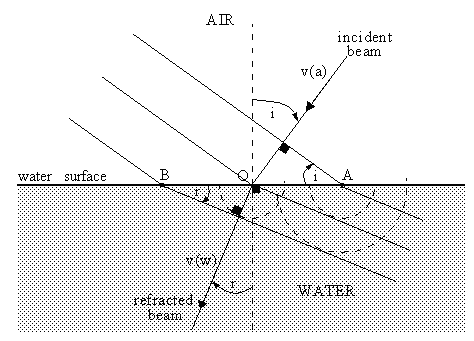# Refraction of Light by Water

When a light beam strikes a plane interface between two media having different optical properties part of it is reflected and part is transmitted. The transmitted beam is refracted, or bent away from the direction of the incident beam. To explain this phenomenon one must resort to the wave theory of light, wherein light is an electromagnetic disturbance consisting of mutually perpendicular oscillating electric and magnetic fields, both of which are perpendicular to the direction of propagation. The theory predicts that the incident, reflected, and refracted beams, as well as the normal to the interface, are all coplanar.In which direction do the wavefronts travel in the water? In the figure, point A lies at the intersection of a line of incident wave crests and the surface of the water. It must also lie on a line of refracted wave crests. Otherwise, the electrons at A would be required to simultaneously perform two different kinds of oscillations, which, of course, they do not. Lines of crests must therefore be continuous at the surface of the water. Accordingly, as the wavefront in air advances by one wavelength v(a)T (speed times period) and the intersection point A moves to point O, the corresponding wavefront in water must also move one wavelength v(w)T in a manner that maintains a match of wave crests at points A and O, as shown.

sin(i) = v(a)T / AO

sin(r) = v(w)T / BO

sin(i)/v(a) = sin(r)/v(w)

n(a)sin(i) = n(w)sin(r)

sin(r) = 0.75*sin(i)